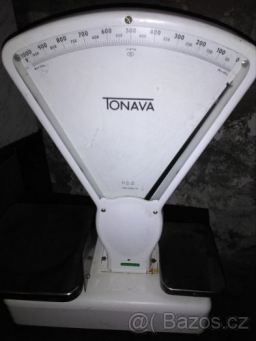# A person

A person buys 10 kg of commodity A at a rate of 2 kg per dollar, 20 kg of commodity b at a rate of 5 kilograms per dollar, and 30 kg of commodity C at a rate of 10 kg per dollar. Find the average price kg per dollar.

p =  5 kg/USD

### Step-by-step explanation:Did you find an error or inaccuracy? Feel free to write us. Thank you!

Tips for related online calculators
Looking for help with calculating arithmetic mean?
Looking for calculator of harmonic mean?
Looking for a statistical calculator?
Need help calculating sum, simplifying, or multiplying fractions? Try our fraction calculator.

#### Grade of the word problem:

We encourage you to watch this tutorial video on this math problem: